# Implementation of Essential Boundary Conditions¶

The essential boundary conditions can be applied in several ways. Here we describe the implementation used in SfePy.

## Motivation¶

Let us solve a linear system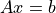with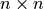matrix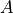with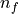values in the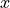vector known. The known values can be for example EBC values on a boundary, ifcomes from a PDE discretization. If we put the known fixed values into a vector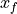, that has the same size as, and has zeros in positions that are not fixed, we can easily construct a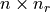matrix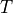that maps the reduced vector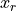of size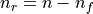, where the fixed values are removed, to the full vector: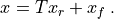With that the reduced linear system with a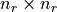can be formed: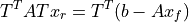that can be solved by a linear solver. We can see, that the (non-zero) known values are now on the right-hand side of the linear system. When the known values are all zero, we have simply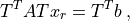which is convenient, as it allows simply throwing away the A and b entries corresponding to the known values already during the finite element assembling.

## Implementation¶

All PDEs in SfePy are solved in a uniform way as a system of non-linear equationswhereis the nonlinear function andthe vector of unknown DOFs. This system is solved iteratively by the Newton method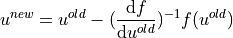until a convergence criterion is met. Each iteration involves solution of the system of linear equations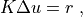where the tangent matrixand the residual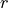are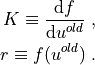ThenIf the initial (old) vectorcontains the values of EBCs at correct positions, the increment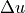is zero at those positions. This allows us to assemble directly the reduced matrix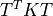, the right-hand side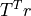, and ignore the values of EBCs during assembling. The EBCs are satisfied automatically by applying them to the initial guess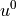, that is given to the Newton solver.

### Linear Problems¶

For linear problems we haveand so the Newton method converges in a single iteration: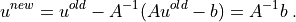### Evaluation of Residual and Tangent Matrix¶

The evaluation of the residualas well as the tangent matrixwithin the Newton solver proceeds in the following steps:

• The EBCs are applied to the full DOF vector.

• The reduced vectoris passed to the Newton solver.

• Newton iteration loop:

• Evaluation of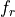or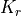:

1.is reconstructed from;

2. local element contributions are evaluated using;

3. local element contributions are assembled intoor- values corresponding to fixed DOF positions are thrown away.

• The reduced system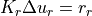is solved.

• Solution is updated: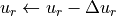.

• The loop is terminated if a stopping condition is satisfied, the solver returns the final.

• The finalis reconstructed from.